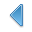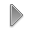Navigation: Realtest Script Language > Syntax Element Details > YIntCategory

Multi-Bar Functions

Description

Linear regression y-intercept

Syntax

YInt(expr, {expr2,} count)

Parameters

expr - data series formula (Y values)

expr2 - optional second data series formula (X values -- a linear series from 1 to count is used if omitted)

count - lookback period

Notes

Calculates the y-intercept of a linear regression of expr evaluated for the previous count bars.